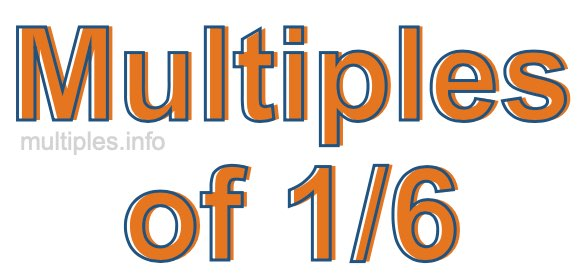Multiples of 1/6We define the multiples of 1/6 as the product of any integer multiplied by 1/6. Therefore, to create a list of multiples of 1/6 we start by multiplying 1/6 by one, then we multiply 1/6 by two, then we multiply 1/6 by three, and so on.

To multiply a fraction by an integer, we leave the denominator alone and multiply the numerator by the integer. It is impossible to give you a list of all multiples of 1/6 because the list goes on forever. However, here is the math to calculate the beginning list of multiples of 1/6.

1/6 × 1 = 1/6

1/6 × 2 = 2/6

1/6 × 4 = 3/6

1/6 × 4 = 4/6

1/6 × 5 = 5/6

1/6 × 6 = 6/6

1/6 × 7 = 7/6

1/6 × 8 = 8/6

1/6 × 9 = 9/6

1/6 × 10 = 10/6

1/6 × 11 = 11/6

1/6 × 12 = 12/6

We have simplified the fractions above for you. Below are the multiples of 1/6 in their lowest possible terms:

1/6 = 1/6

2/6 = 1/3

3/6 = 1/2

4/6 = 2/3

5/6 = 5/6

6/6 = 1

7/6 = 1 1/6

8/6 = 1 1/3

9/6 = 1 1/2

10/6 = 1 2/3

11/6 = 1 5/6

12/6 = 2

As we stated above, the list of multiples of 1/6 goes on forever because the product of 1/6 multiplied by any whole number (integer) is a multiple of 1/6. You can also create a list of multiples of 1/6 if you keep adding 1/6 like this:

1/6
1/6 + 1/6 = 2/6
1/6 + 1/6 + 1/6 = 3/6
1/6 + 1/6 + 1/6 + 1/6 = 4/6

Again, the list can go on forever, because you can continue adding 1/6 to the list. Therefore, the list of multiples of 1/6 is ∞ (infinite).

Multiples of Fractions
Do you need the multiples of another fraction? Enter a another fraction below to get the multiples of that fraction.

/

Multiples of 1/7
Go here for the next fraction on our list that we have multiples for.